# Electric Field of Point Charge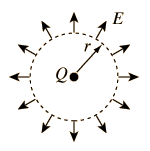The electric field of a point charge Q can be obtained by a straightforward application of Gauss' law. Considering a Gaussian surface in the form of a sphere at radius r, the electric field has the same magnitude at every point of the sphere and is directed outward. The electric flux is then just the electric field times the area of the sphere.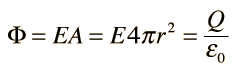The electric field at radius r is then given by: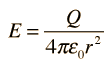If another charge q is placed at r, it would experience a force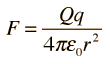so this is seen to be consistent with Coulomb's law.

 Charged conducting sphere Sphere of uniform charge
 Fields for other charge geometries
Index

Electric field concepts

 HyperPhysics***** Electricity and Magnetism R Nave
Go Back

# Electric Field of Conducting Sphere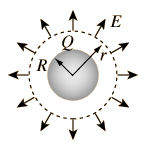The electric field of a conducting sphere with charge Q can be obtained by a straightforward application of Gauss' law. Considering a Gaussian surface in the form of a sphere at radius r > R , the electric field has the same magnitude at every point of the surface and is directed outward. The electric flux is then just the electric field times the area of the spherical surface.The electric field is seen to be identical to that of a point charge Q at the center of the sphere. Since all the charge will reside on the conducting surface, a Gaussian surface at r< R will enclose no charge, and by its symmetry can be seen to be zero at all points inside the spherical conductor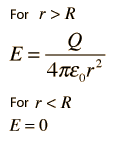Point charge Sphere of uniform charge
 Potential of conducting sphere
 Fields for other charge geometries
Index

Electric field concepts

 HyperPhysics***** Electricity and Magnetism R Nave
Go Back

# Electric Field: Sphere of Uniform Charge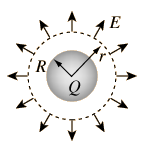The electric field of a sphere of uniform charge density and total charge charge Q can be obtained by applying Gauss' law. Considering a Gaussian surface in the form of a sphere at radius r > R, the electric field has the same magnitude at every point of the surface and is directed outward. The electric flux is then just the electric field times the area of the spherical surface.The electric field outside the sphere (r > R)is seen to be identical to that of a point charge Q at the center of the sphere.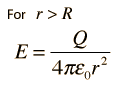For a radius r < R, a Gaussian surface will enclose less than the total charge and the electric field will be less. Inside the sphere of charge, the field is given by: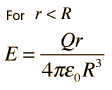Show
 Point charge Charged conducting sphere
 Fields for other charge geometries
Index

Electric field concepts

 HyperPhysics***** Electricity and Magnetism R Nave
Go Back

# Inside a Sphere of Charge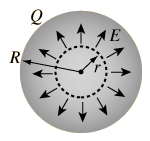The electric field inside a sphere of uniform charge is radially outward (by symmetry), but a spherical Gaussian surface would enclose less than the total charge Q. The charge inside a radius r is given by the ratio of the volumes: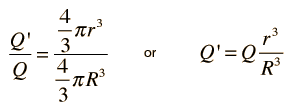The electric flux is then given by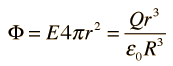and the electric field is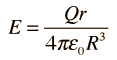Note that the limit at r= R agrees with the expression for r >= R. The spherically symmetric charge outside the radius r does not affect the electric field at r. It follows that inside a spherical shell of charge, you would have zero electric field.

 Field outside charged sphere
Index

Electric field concepts

 HyperPhysics***** Electricity and Magnetism R Nave
Go Back